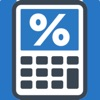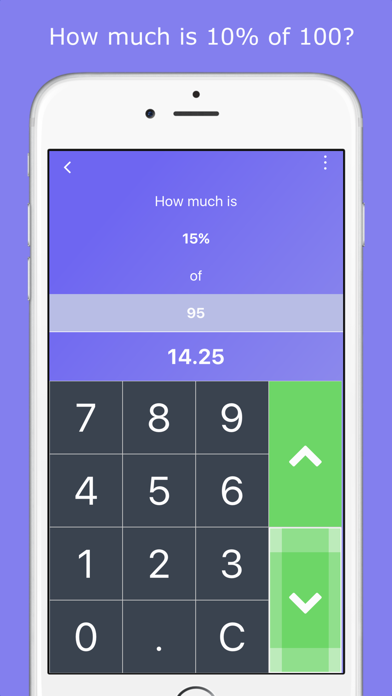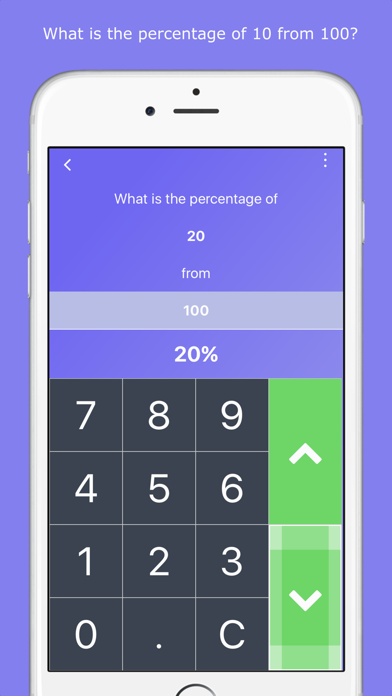## Easy Percentage Calculator - Lumos Educational App Store3.416671
Price -Free
\$0

#### DESCRIPTION:

Our calculator has made it easier than ever before to calculate any percentage you need. You don't have to use any formulas – you just type in the numbers and calculate. Examples: How much is 25% of 110? Or what is the percentage for 11 from 20? You can use our calculator not only for easy and everyday calculations, but also for complex ones. Here is the full list of calculations our calculator can help you with: - What is the percentage for 10 from 100? - How much is 10% of 100? - What is the total amount if 10 equals 20% of the total amount? - What is the change in percentage if the amount

#### OVERVIEW:

Easy Percentage Calculator is a free educational mobile app By RedVok Software.It helps students practice the following standards .

This page not only allows students and teachers download Easy Percentage Calculator but also find engaging Sample Questions, Videos, Pins, Worksheets, Books related to the following topics.

Developer: RedVok Software

Developer URL: NA

Software Version: 2.0

Category: Utilities

Release Date: 2010-09-11T20:42:38Z#### Are you the Developer?EdSearch WebSearch